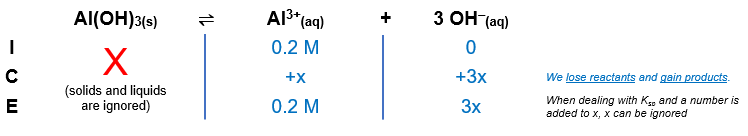# Problem: The Ksp of Al(OH)3 is 2 X 10-32. At what pH will a 0.2-M Al 3+ solution begin to show precipitation of Al(OH)3?

###### FREE Expert Solution

Al(OH)3(s) Al3+(aq) + 3 OH(aq)$\mathbf{2}\mathbf{.}\mathbf{0}\mathbf{×}{\mathbf{10}}^{\mathbf{-}\mathbf{32}}\mathbf{=}\mathbf{\left(}\mathbf{0}\mathbf{.}\mathbf{2}\mathbf{\right)}\mathbf{\left(}\mathbf{27}{\mathbf{x}}^{\mathbf{3}}\mathbf{\right)}\phantom{\rule{0ex}{0ex}}\frac{\mathbf{2}\mathbf{.}\mathbf{0}\mathbf{×}{\mathbf{10}}^{\mathbf{-}\mathbf{32}}}{\mathbf{5}\mathbf{.}\mathbf{4}}\mathbf{=}\frac{\overline{)\mathbf{5}\mathbf{.}\mathbf{4}}{\mathbf{x}}^{\mathbf{3}}}{\overline{)\mathbf{5}\mathbf{.}\mathbf{4}}}\phantom{\rule{0ex}{0ex}}\sqrt[\mathbf{3}]{\mathbf{3}\mathbf{.}\mathbf{7037}\mathbf{×}{\mathbf{10}}^{\mathbf{-}\mathbf{33}}}\mathbf{=}\sqrt[\mathbf{3}]{{\mathbf{x}}^{\mathbf{3}}}$

x = 1.5472x10-11

96% (10 ratings)###### Problem Details

The Ksp of Al(OH)3 is 2 X 10-32. At what pH will a 0.2-M Al 3+ solution begin to show precipitation of Al(OH)3?